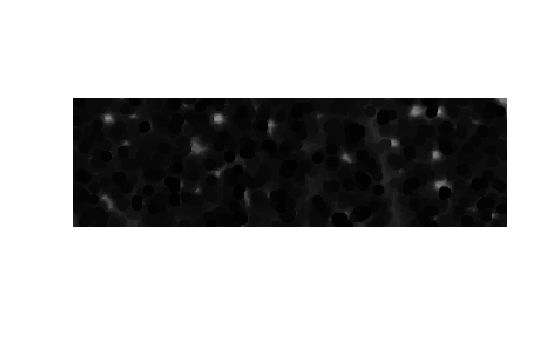Documentation

This is machine translation

Mouseover text to see original. Click the button below to return to the English version of the page.

imreconstruct

Morphological reconstruction

Syntax

``J = imreconstruct(marker,mask)``
``J = imreconstruct(marker,mask,conn)``

Description

example

````J = imreconstruct(marker,mask)` performs morphological reconstruction of the image `marker` under the image `mask`, and returns the reconstruction in `J`. The elements of `marker` must be less than or equal to the corresponding elements of `mask`. If the values in `marker` are greater than corresponding elements in `mask`, then `imreconstruct` clips the values to the `mask` level before starting the procedure.You optionally can perform morphological reconstruction of 2-D images using a GPU (requires Parallel Computing Toolbox™). For more information, see Image Processing on a GPU.```
````J = imreconstruct(marker,mask,conn)` performs morphological reconstruction with the specified connectivity, `conn`. ```

Examples

collapse all

Read a grayscale image and display it.

```I = imread('snowflakes.png'); figure imshow(I)```Adjust the contast of the image to create the mask image and display results.

```mask = adapthisteq(I); figure imshow(mask)```Create a marker image that identifies high-intensity objects in the image using morphological erosion and display results.

```se = strel('disk',5); marker = imerode(mask,se); imshow(marker)```Perform morphological opening on the mask image, using the marker image to identify high-intensity objects in the mask. Display results.

```obr = imreconstruct(marker,mask); figure imshow(obr,[])```Read a logical image into workspace and display it. This is the mask image.

```mask = imread('text.png'); figure imshow(mask)```Create a marker image that identifies the object in the image you want to extract through segmentation. For this example, identify the "w" in the word "watershed".

```marker = false(size(mask)); marker(13,94) = true;```

Perform segmentation of the mask image using the marker image.

```im = imreconstruct(marker,mask); figure imshow(im)```Read mask image and create `gpuArray`.

```mask = gpuArray(imread('text.png')); figure, imshow(mask)```

Create marker image `gpuArray`.

```marker = gpuArray.false(size(mask)); marker(13,94) = true; ```

Perform the segmentation and display the result.

```J = imreconstruct(marker,mask); figure, imshow(J)```

Input Arguments

collapse all

Input image, specified as a numeric or logical array.

To perform the morphological reconstruction using a GPU, specify `marker` as a `gpuArray` that contains a 2-D numeric or logical matrix. `imreconstruct` does not support RGB images and 3-D images on a GPU.

Example: `se = strel('disk',5); marker = imerode(mask,se);`

Example: ```marker = gpuArray(imread('text.png'));```

Data Types: `single` | `double` | `int8` | `int16` | `int32` | `int64` | `uint8` | `uint16` | `uint32` | `uint64` | `logical`

Mask image, specified as a numeric or logical array of the same size and data type as `marker`.

To perform the morphological reconstruction using a GPU, specify `mask` as a `gpuArray` that contains a 2-D numeric or logical matrix. `imreconstruct` does not support RGB images and 3-D mask images on a GPU.

Example: `mask = imread('text.png');`

Example: ```mask = gpuArray(imread('text.png'));```

Data Types: `single` | `double` | `int8` | `int16` | `int32` | `int64` | `uint8` | `uint16` | `uint32` | `uint64` | `logical`

Pixel connectivity, specified as one of the values in this table. The default connectivity is `8` for 2-D images, and `26` for 3-D images.

Value

Meaning

Two-Dimensional Connectivities

4-connected

Pixels are connected if their edges touch. The neighborhood of a pixel are the adjacent pixels in the horizontal or vertical direction.8-connected

Pixels are connected if their edges or corners touch. The neighborhood of a pixel are the adjacent pixels in the horizontal, vertical, or diagonal direction.Three-Dimensional Connectivities

6-connected

Pixels are connected if their faces touch. The neighborhood of a pixel are the adjacent pixels in:

• One of these directions: in, out, left, right, up, and down18-connected

Pixels are connected if their faces or edges touch. The neighborhood of a pixel are the adjacent pixels in:

• One of these directions: in, out, left, right, up, and down

• A combination of two directions, such as right-down or in-up26-connected

Pixels are connected if their faces, edges, or corners touch. The neighborhood of a pixel are the adjacent pixels in:

• One of these directions: in, out, left, right, up, and down

• A combination of two directions, such as right-down or in-up

• A combination of three directions, such as in-right-up or in-left-downFor higher dimensions, `imreconstruct` uses the default value `conndef(ndims(marker),'maximal')`.

Connectivity can also be defined in a more general way for any dimension by specifying a 3-by-3-by- ... -by-3 matrix of `0`s and `1`s. The `1`-valued elements define neighborhood locations relative to the center element of `conn`. Note that `conn` must be symmetric about its center element. See Specifying Custom Connectivities for more information.

Data Types: `double` | `logical`

Output Arguments

collapse all

Reconstructed image, returned as a numeric or logical array, depending on the input image, that is the same size as the input image.

If the morphological reconstruction is performed using a GPU, then `J` is returned as a `gpuArray` that contains a numeric or logical matrix.

Algorithms

`imreconstruct` uses the fast hybrid grayscale reconstruction algorithm described in .

 Vincent, L., "Morphological Grayscale Reconstruction in Image Analysis: Applications and Efficient Algorithms," IEEE Transactions on Image Processing, Vol. 2, No. 2, April, 1993, pp. 176-201.